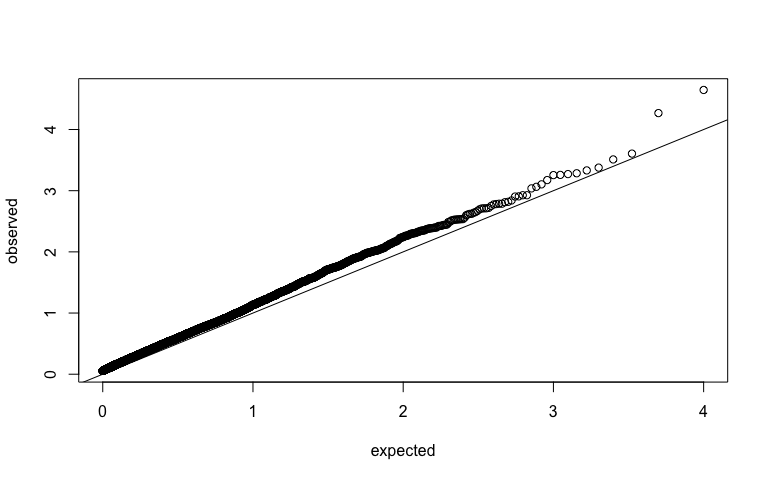# GEint Tutorial

#### 2017-01-06

GEint is designed to (1) provide results about the bias of fitted regression covariates in misspecified Gene-Environment Interaction (GxE) models and (2) offer the novel Bootstrap Inference with Corrected Sandwich (BICS) procedure for performing testing in GxE models. For the statistical details, see:

Testing for Gene-Environment Interaction under Misspecification of the Environment, Submitted, Sun, Carroll, Christiani, and Lin (2017)

This short vignette is designed to show you the main functionality of GEint. Specifically, it will cover:

• An introduction to the types of problems we attempt to solve.
• How to use GE_bias(), GE_bias_normal_squaredmis(), and GE_nleqlv() to perform bias analysis of fitted regression coefficients in GxE interaction models.
• How to use BICS() to perform inference in GxE interaction models.

## Introduction - Background and statistical setting

A Genome-Wide Environment Interaction Study (GWEIS) usually involves a large number of genetic markers (d~500,000), a single environmental exposure, and a single outcome. For each of these markers, we typically fit a separate regression model for the outcome. For each $$j$$=1,…,d, the $$j$$th marker, the environment, and a ($$j$$th marker)-environment interaction term is included in the linear predictor (possibly other covariates as well). A major issue in conducting (GWEIS) is that $$d$$ p-values from testing the interaction terms often show a large departure from uniformity, while p-values from a standard GWAS on the same data (fitting a model without the interaction term and testing only for the effect of the genetic marker) appear to be completely uniform. For examples, GWEIS p-values may look like:We show in our paper that misspecification of the GWEIS model can lead to biased estimates of the interaction coefficient, which is a possible cause of nonuniform p-values like those seen above. This bias can be calculated analytically for linear regression models. Another possible cause of the nonuniform p-values is bias in the standard error estimate for the regression coefficient. In the paper we show that the commonly proposed ‘sandwich’ standard error estimate can be anticonservative in moderate samples. We propose BICS to correct the issue.

Specifically in the paper and in this package we assume that the true model for the outcome is:

$E[Y_{i}] = \beta_{0} + \beta_{G}*G_{i} + \beta_{E}*f(E_{i}) + \beta_I*G_{i}*h(E)_{i} + \mathbf{Z}_{i}^{T}\boldsymbol{\beta}_{Z} + \mathbf{M}_{i}^{T}\boldsymbol{\beta}_{M}$

while the fitted model is:

$E[Y_{i}] = \alpha_{0} + \alpha_{G}*G_{i} + \alpha_{E}*E_{i} + \alpha_I*G_{i}*E_{i} + \mathbf{Z}_{i}^{T}\boldsymbol{\alpha}_{Z} + \mathbf{W}_{i}^{T}\boldsymbol{\alpha}_{W}$

with $$f()$$ and $$h()$$ being known functions, and $$\mathbf{Z}_{i}$$ and $$\mathbf{W}_{i}$$ being vectors of length $$p$$ and $$q$$ respectively.

## Bias Analysis

To get an exact, analytical solution for the asymptotic values of $$\alpha_{0},\alpha_{G}...,\boldsymbol{\alpha}_{W}$$, we obviously need to know the corresponding values of $$\beta_{0},\beta_{G}...,\boldsymbol{\beta}_{M}$$ in the true model. We also need to know a number of expectations of the form $$E[G*E]$$ and a number of higher order moments such as $$E[G*G*h(E)]$$. Once we know these terms, we can pass them all to GE_bias() and get the exact solution for the fitted values. Since there are a lot of terms, we provide the helper function GE_enumerate_inputs() which tells you the exact terms needed as well as the order to input them.

However, many researchers may not have a good idea of how to calculate/estimate so many expectations and higher order moments. To simplify the calculations, we offer the function GE_bias_normal_squaredmis(). This function makes some strong assumptions, but in exchange, it allows the user to input a much smaller vector of correlations, and then the function will calculate the rest of the terms. Specifically we assume (1) that the two minor alleles of the genetic term are generated by thresholding two independent normal random variables. We also assume that (2) the two independent normals from (1) as well as the other fitted covariates E,Z,W have a joint multivariate normal distribution with common mean 0 and marginal variance 1. Finally we assume that (3) $$f(x)=h(x)=x^{2}$$ and that $$M_{j}=W_{j}^{2}$$ for all $$j=1,...,q$$. These assumptions correspond to the case where the researcher has standardized all their covariates to have mean 0 and variance 1 (expect for G, which is centered at 0 but does not have unit variance), and the covariates appear to be approximately normally distributed. GE_bias_normal_squaredmis() will also output the calculated higher order terms for use with GE_bias() in case you would like to see how those terms look.

An simple example follows:

library(GEint)
beta_list <- list(1, 1, 1, 0, c(1,1), 1)
rho_list <- list(0.1, c(0.1, 0.1), c(0.1,0.1), 0.1, 0.1, c(0.1, 0.1))
prob_G <- 0.3
cov_Z <- 0.1
cov_W <- NULL
normal_assumptions <- GE_bias_normal_squaredmis(beta_list=beta_list, rho_list=rho_list, prob_G=prob_G, cov_Z=cov_Z)

Note that in this example $$Z$$ has dimension 2 and $$W$$ has dimension 1. We can use the higher order moments calculated in GE_bias_normal_squaredmis() as input for GE_bias() to check our work:

cov_list <- normal_assumptions$cov_list cov_mat_list <- normal_assumptions$cov_mat_list
mu_list <- normal_assumptions$mu_list HOM_list <- normal_assumptions$HOM_list
no_assumptions <- GE_bias(beta_list, cov_list, cov_mat_list, mu_list, HOM_list)

# The results should match:
unlist(no_assumptions)
##   2.948581945  0.988973537 -0.002134513  0.514180555  0.997865487
##   0.997865487 -0.002134513
unlist(normal_assumptions\$alpha_list)
##   2.948581945  0.988973537 -0.002134513  0.514180555  0.997865487
##   0.997865487 -0.002134513

They match! The results are presented in the order $$\alpha_{0}, \alpha_{G}, \alpha_{E}, \alpha_{I}, \boldsymbol{\alpha}_{Z}, \boldsymbol{\alpha}_{W}$$.

## Bootstrap Inference with Corrected Sandwich (BICS)

Even if the regression coefficient $$\alpha_{I}$$ is asymptotically unbiased, we may still see non-uniform p-values if our standard error estimate is biased in finite samples. Thus we introduce the BICS estimator. To apply BICS almost as simple as a standard lm() call. Just give the function your outcome and design matrix. To apply BICS genome-wide, simply adjust the design matrix for each new $$G$$ term and then run BICS again.

set.seed(100)
n <- 500
Y_continuous <- rnorm(n=n)
Y_binary <- rbinom(n=n, size=1, prob=0.5)
E <- rnorm(n=n)
G <- rbinom(n=n, size=2, prob=0.3)
design_mat <- cbind(1, G, E, G*E)

GE_BICS(outcome=Y_continuous, design_mat=design_mat, desired_coef=4, outcome_type='C')
##  0.179223
GE_BICS(outcome=Y_binary, design_mat=design_mat, desired_coef=4, outcome_type='D')
##  0.868443